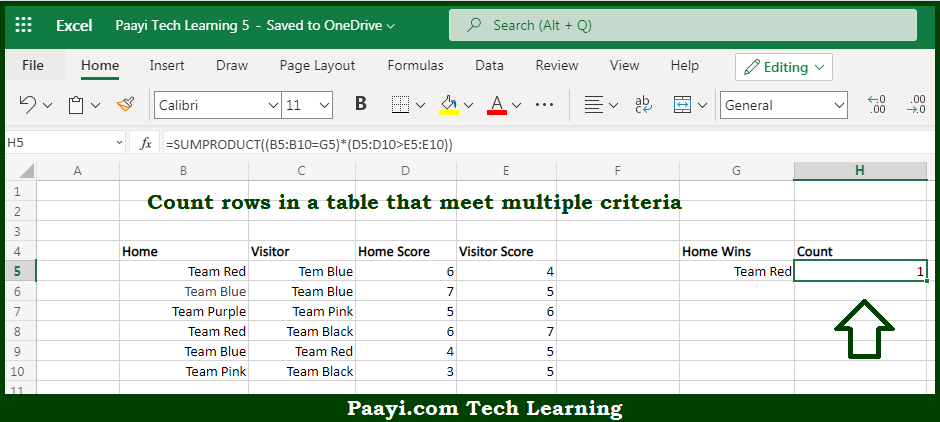# Learn How to Count if Row Meets Multiple Internal Criteria in Microsoft Excel

Written by | 0 Comments | 599 Views

In this article, you will learn how to COUNT various things in Microsoft Excel using a single or combination of functions and its purpose in Microsoft Excel. You will also get to know how to Count if Row Meets Multiple Internal Criteria & generic formula

Count if Row Meets Multiple Internal Criteria in Microsoft Excel

The main purpose of this formula is to count rows in a given table that meets multiple internal criteria. Here we will learn how to count rows that meet multiple internal criteria in Microsoft Excel. That implies, with the help of a formula based on the SUMPRODUCT function you can able to count rows in a table that meets the multiple criteria, some of those criteria may depend on logical tests that work at the row level. So, with the help of this formula, you can able to count rows in a given table that meets multiple internal criteria.

General Formula to Count if Row Meets Multiple Internal Criteria

=SUMPRODUCT((logical1)*(logical2))

The Explanation for the Count if Row Meets Multiple Internal CriteriaSo we know that with the help of the given formula above you can able to count rows in a given table that meets multiple internal criteria. Here we will learn how to count rows that meet multiple internal criteria in Microsoft Excel. As we know that, the SUMPRODUCT's main purpose is to work smoothly with arrays. It multiplies corresponding elements in two or more arrays and sums the resulting products. That is why you can use the SUMPRODUCT function to process arrays that result from criteria being applied to a range of cells. It should be noted that the result of such operations will be arrays, which the SUMPRODUCT function can handle efficiently. By using the multiplication operator to multiply the two arrays together, Excel will automatically coerce logical values to ones and zeros So, with the help of this formula, you can able to count rows in a given table that meets the multiple internal criteria.